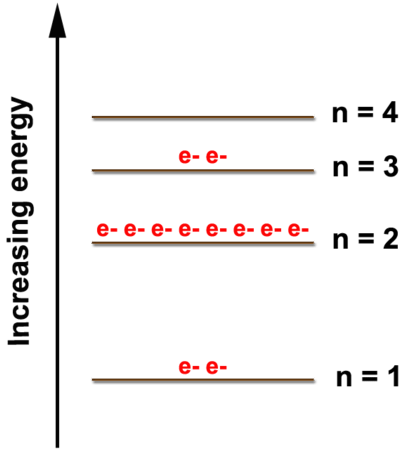# 2.4: Electron Arrangements

•• Contributed by Allison Soult
• Senior Lecturer (Chemistry) at University of Kentucky

Learning Outcomes

• Determine the energy levels of electrons for the first 20 elements.
• Explain the relevance of valence electrons in chemical processes.
• Identify the number of valence electrons in an element.
• Describe the stability of an atom as a result of following the octet rule.

The structure of the atom was discussed in the previous unit and now we will focus on the role that electrons play in the formation of compounds. Regardless of the type of compound or the number of atoms or electrons involved, it is the electrons of those atoms that interact to form a compound.

## Electron Arrangement

Electrons are not randomly arranged in an atom and their position within the atom can be described using electron arrangements, which are a simplified version of electron configurations. For each element of interest, we look at the number of electrons in a single atom and then determine how those electrons are arranged based on the atomic model. The main idea behind electron arrangements is that electrons can only exist at certain energy levels. By understanding the energy levels of electrons in an atom, we can predict properties and understand behavior of the atom.

As shown in the figure below, there are multiple energy levels where electrons can be found. As the energy level increases, the energy difference between them decreases. A maximum of two electrons can be found in the $$n=1$$ level; eight electrons can be in the $$n=2$$ level. Although the $$n=3$$ and $$n=4$$ levels show only eight electrons in this diagram, those energy levels can hold more but not until we start looking at the transition metals. We will only be concerned with the electron arrangements of elements through calcium $$\left( Z = 20 \right)$$ so we will put a maximum of eight electrons in the $$n=3$$ level and two in the $$n=4$$ level.Figure $$\PageIndex{1}$$: Energy levels of electrons.

Example $$\PageIndex{1}$$

What is the electron arrangement of oxygen?

Solution

Oxygen has eight electrons. The first two electrons will go in the $$n=1$$ level. Two is the maximum number of electrons for the level so the other electrons will have to go in a higher energy level. The $$n=2$$ level can hold up to eight electrons so the remaining six electrons will go in the $$n=2$$ level. The electron arrangement of oxygen is (2, 6).

Example $$\PageIndex{2}$$

What is the electron arrangement of chlorine?

Solution

Chlorine has 17 electrons. The first two electrons will go in the $$n=1$$ level. Two is the maximum number of electrons for the level so the other electrons will have to go in higher energy levels. The $$n=2$$ level can hold up to eight electrons so the next 8 electrons will go in the $$n=2$$ level. The remaining 7 electrons can go in the $$n=3$$ level since it holds a maximum of 8 electrons. The electron arrangement of chlorine is (2, 8, 7).

The electron arrangement also provides information about the number of valence electrons. The valence electrons are the electrons in the highest energy level and the ones involved in ion and bond formation. Knowing the number of valence electrons will allow us to predict how a particular element will interact with other elements. Electrons in lower energy levels are called the core electrons.

Let's look at the figure below which shows the electron diagram for magnesium and its 12 electrons. The first two electrons are found in the $$n=1$$ energy level, the next eight electrons are found in the $$n=2$$ level, and the remaining two electrons are found in the $$n=3$$ level. The electrons always fill the lowest energy levels available until that level is filled, then electrons fill the next energy level until it is filled. This continues for all of the electrons in an atom. We can show the electron arrangement as (2, 8, 2) representing the electrons in the $$n=1$$, $$n=2$$, and $$n=3$$ levels, respectively.Figure $$\PageIndex{2}$$: Electron diagram for magnesium.

The electron arrangement also shows the number of valence electrons which is two for magnesium because there are two electrons in the $$n=3$$ energy level which is the highest occupied energy level for magnesium. This corresponds to the $$2+$$ charge formed when magnesium forms an ion. It is willing to lose 2 electrons so that it has the same electron arrangements as the nearest noble gas, which is neon (2, 8). Atoms will gain or lose electrons to look like the nearest noble gas because the noble gases are unreactive due to the stability of having eight electrons in the highest energy level. This desire of atoms to have eight electrons in their outermost shell is known as the octet rule.

Example $$\PageIndex{3}$$

What is the electron arrangement of aluminum? How many valence electrons does it have?

Solution

Aluminum has 13 electrons so it will have the electron arrangement (2, 8, 3) which represents two electrons in the $$n=1$$ energy level, eight electrons in the $$n=2$$ level, and three electrons in the $$n=3$$ level. Aluminum has three valence electrons (indicated by the three electrons in the $$n=3$$ level).

Example $$\PageIndex{4}$$

How many valence electrons does chlorine have? How many electrons will chlorine gain or lose to form an ion?

Solution

Chlorine has 7 electrons in its valence shell. To meet the octet rule, it must either gain one electron or lose seven electrons. Gaining one is easier than losing seven so it will gain one electron to have a total of eight electrons when it forms an ion (i.e. charged particle).